# Errata

Special thanks go to Frank Schultz for numerous suggestions for comments and corrections.

# General

The usage of the wavenumber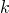is not consistent throughout the book. In many placeshas to be replaced by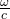since these two expressions are not fully equivalent: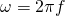can become negative (such as in a Fourier transform, e.g., Eq. (B.4)) whereas, the length of the wave vector, is always non-negative. Eq. (2.3) equates the square ofandfor the same reason.

Examples for expressions to which the replacement should be applied are Eq. (1.3) and (A.3). Note that the trace wavenumbers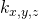can indeed become negative.

Another aspect to point out is the fact that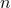is indeed the order of a Bessel or Hankel function. However, when it comes to associated Legendre functions and surface spherical harmonics, thenis termed the degree and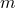the order.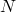or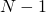may still be termed the maximum order of an expansion because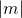will never exceed(or, respectively).

# Page 7, Fig. 1.3

ITU-R BS.775 is a recommendation, not a standard.

# Page 28, Fig. 2.4

Note that all subfigures are normalized such that the maximum is 1. Multiply (a) by 0.28, (b) by 0.35, (c) by 0.63, and (d) by 0.39 to undo the normalization.

# Pages 31-32, Eq. (2.37)

The factor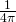is missing on the left-hand side of Eq. (2.37a) and (2.37b).

# Page 39, Eq. (2.44)

The factor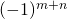has to be removed from Eq. (2.44). (It turns the expansion upside down.) In order to normalize the look direction to 1, multiply by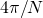. (Thanks to Mark R. P. Thomas for this hint.)

# Page 44, Fig. (2.13)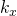shown on the horizontal axes in both subfigures is given in rad / m and not in rad.

# Page 49, Sec. 2.3.1

I left out the letter “i” in several instances. The gentleman was called Dirichlet (as in the title of the section).

# Page 49, Sec. 2.3.2

From Eq. (2.62) it is clear that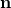is of unit length. This is not mentioned in the text (but assumed throughout the book).

# Page 51, Eq. (2.67)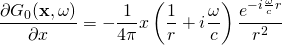Note that Eq. (2.67) represents the directional gradient of a monopole source located in the coordinate origin.

# Page 62, Eq. (3.15)

I reconsidered the formulation of Eq. (3.15), which is kind of sloppy in the book. A nicer representation is

(1)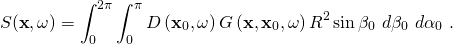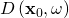denotes the driving function of the secondary source located at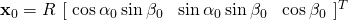.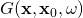denotes the spatio-temporal transfer function of the secondary source at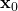. The proof that (1) does indeed constitute a spherical convolution follows below.

Spherical convolution of two functions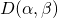and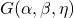is defined in (Driscoll and Healy 1994) as

(2)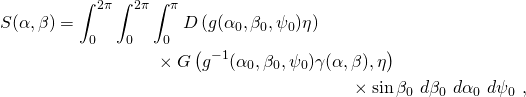whereby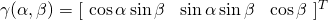represents a location on the unit sphere and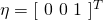denotes the north pole of the latter. Using Euler matrix representation of rotation, the rotation matrix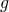can be written as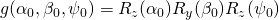, whereby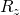and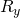are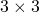matrices that represent counterclockwise rotations about the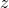and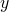axes, respectively.

The convolution theorem

(3)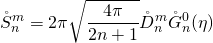holds. Eq. (2) can be rewritten to be (Rafaely 2004)

(4)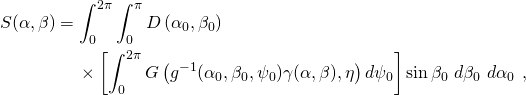where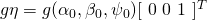reduces to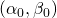. Assuming that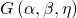is invariant with respect to rotation about theaxis, which is the case, e.g., whenrepresents a monopole source at the north pole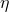of the sphere, then the inner integral in (4) evaluates to

(5)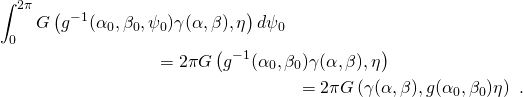The latter equality only holds under free-field conditions, i.e., when a rotation of the coordinate system is equal to an according rotation of the source arrangement in opposite direction. Eq. (3.15) can thus be transformed to (3.17) under the given assumptions, whereby actually the factor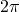has to be removed from (3.17) (and subsequent equations).

Above derivation also points out why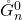in (3) (and (3.17), respectively) represents the coefficients of the secondary source at the north pole as it is stated in the third bullet point on page 63.

# Page, 68, Eq. (3.27)

The summation over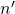has to go from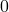to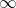.

# Page 91

The large argument approximation of the Hankel function is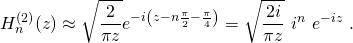# Page 105, Eq. (3.101)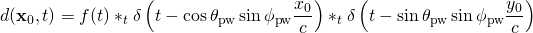# Page 170, Fig. 4.42

The sound field depicted in Fig. 4.42 exhibits a frequency of 1300 Hz and not 1000 Hz as stated in the caption.

# Page 230, Sec. 5.7.1

The solution presented in this section is only valid if the source moves along the x-axis. Look here for an extension to arbitrary straight trajectories.

# Page 231, Eq. (5.55)

The retarded time is actually defined as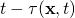, i.e., it is the time that the sound source experiences with respect to the input signal.

# Page 231, Eq. (5.56)

There is an equals sign missing at the beginning of the second line of Eq. (5.56).

# Page 233, Eq. (5.62)

The first case is valid for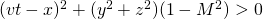and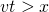.